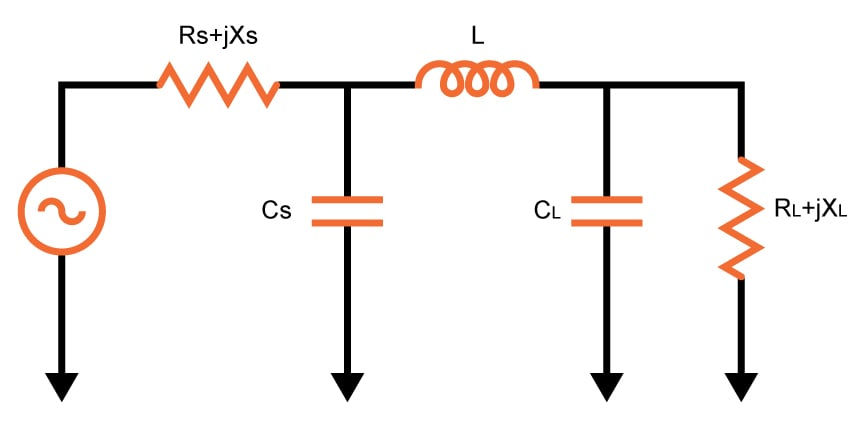# Pi-Match Impedance Matching Calculator

## This calculator is designed to compute the parameters required to design a Pi-Match Impedance Circuit

Ohm
Ohm
Ohm
Ohm
Ohm

### Overview

The Pi match impedance matching circuit is used to match the impedance between two points, usually a source and a load. The circuit got its name because the inductor and the capacitor form a Pi symbol (see schematic below).

This calculator will help you determine the correct values for the inductor and capacitor in a Pi match impedance matching circuit. The required parameters are the signal (or source) frequency, the impedance of the source  and the impedance of the load. You can also specify if the circuit will pass or block the direct current (DC). The inductor quality factor is also computed.### Equations

For Pass DC Current:

$$Z_{input}=\left \{ \left [ \left ( R_{L}+jX_{L} \right )//\left ( \frac{1}{j\omega\cdot C_{L}} \right ) \right ]+j\omega\cdot L \right \}//\left ( \frac{1}{j\cdot \omega\cdot C_{s}} \right )$$

For Block DC Current:

$$Z_{input}=\left \{ \left [ \left ( R_{L}+jX_{L} \right )//\left ( j\omega\cdot L_{L} \right ) \right ]+\frac{1}{j\omega\cdot C} \right \}//\left ( j\omega\cdot L_{s} \right )$$

### Applications

Impedance matching is important in electrical engineering because it allows for maximum power transfer between two points. In telephone systems, for example, minimizing echo on long-distance lines is achieved using match impedances. The telephone hybrid coil, where two wires are converted into four wires can also be achieved via matching.

In audio amplifiers, impedances are not matched as it is typical for amplifiers to have output impedances that is lower than the load impedance for better speaker damping. But for higher-power amplifiers, such as those that use vacuum tubes, impedance-changing circuits are used to get a low output impedance in order to better match the amplifier's performance to the load impedance.•ateequeur rahman October 17, 2017

what do this “NaN” mean??

Like.
• R
Robert Keim October 17, 2017

“NaN” stands for “not a number” and indicates that something went wrong in the calculation.

Like.
•Konstadinos Hatzianestis August 30, 2021

What are the conditions that cause the results to be NaN ?

Is this calculator working at all ?

Like.